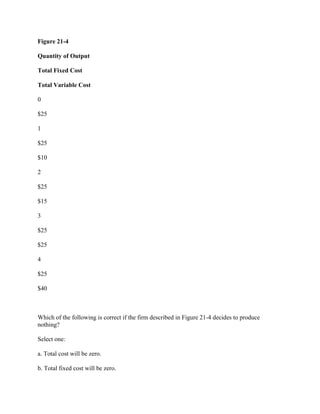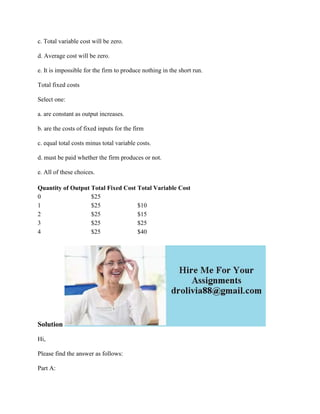Seu SlideShare está sendo baixado. ×

# Figure 21-4 Quantity of Output Total Fixed Cost Total Variable Cost 0.docx

Anúncio
Anúncio
Anúncio
Anúncio
Anúncio
Anúncio
Anúncio
Anúncio
Anúncio
Anúncio
AnúncioCarregando em…3
×

1 de 3 Anúncio

# Figure 21-4 Quantity of Output Total Fixed Cost Total Variable Cost 0.docx

Figure 21-4
Quantity of Output
Total Fixed Cost
Total Variable Cost
0
\$25
1
\$25
\$10
2
\$25
\$15
3
\$25
\$25
4
\$25
\$40
Which of the following is correct if the firm described in Figure 21-4 decides to produce nothing?
Select one:
a. Total cost will be zero.
b. Total fixed cost will be zero.
c. Total variable cost will be zero.
d. Average cost will be zero.
e. It is impossible for the firm to produce nothing in the short run.
Total fixed costs
Select one:
a. are constant as output increases.
b. are the costs of fixed inputs for the firm
c. equal total costs minus total variable costs.
d. must be paid whether the firm produces or not.
e. All of these choices.
Solution
Hi,
Part A:
Option C (Total variable cost will be zero) is the correct answer.
--
Part B:
Option E (All of these) is the correct answer.
Thanks.
.

Figure 21-4
Quantity of Output
Total Fixed Cost
Total Variable Cost
0
\$25
1
\$25
\$10
2
\$25
\$15
3
\$25
\$25
4
\$25
\$40
Which of the following is correct if the firm described in Figure 21-4 decides to produce nothing?
Select one:
a. Total cost will be zero.
b. Total fixed cost will be zero.
c. Total variable cost will be zero.
d. Average cost will be zero.
e. It is impossible for the firm to produce nothing in the short run.
Total fixed costs
Select one:
a. are constant as output increases.
b. are the costs of fixed inputs for the firm
c. equal total costs minus total variable costs.
d. must be paid whether the firm produces or not.
e. All of these choices.
Solution
Hi,
Part A:
Option C (Total variable cost will be zero) is the correct answer.
--
Part B:
Option E (All of these) is the correct answer.
Thanks.
.

Anúncio
Anúncio

### Figure 21-4 Quantity of Output Total Fixed Cost Total Variable Cost 0.docx

1. 1. Figure 21-4 Quantity of Output Total Fixed Cost Total Variable Cost 0 \$25 1 \$25 \$10 2 \$25 \$15 3 \$25 \$25 4 \$25 \$40 Which of the following is correct if the firm described in Figure 21-4 decides to produce nothing? Select one: a. Total cost will be zero. b. Total fixed cost will be zero.
2. 2. c. Total variable cost will be zero. d. Average cost will be zero. e. It is impossible for the firm to produce nothing in the short run. Total fixed costs Select one: a. are constant as output increases. b. are the costs of fixed inputs for the firm c. equal total costs minus total variable costs. d. must be paid whether the firm produces or not. e. All of these choices. Quantity of Output Total Fixed Cost Total Variable Cost 0 \$25 1 \$25 \$10 2 \$25 \$15 3 \$25 \$25 4 \$25 \$40 Solution Hi, Please find the answer as follows: Part A:
3. 3. Option C (Total variable cost will be zero) is the correct answer. -- Part B: Option E (All of these) is the correct answer. Thanks.